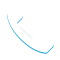### Statistical Analysis Using IBM SPSS Statistics (V26) – 0G51BG CR

Details

Course Code: 0G51BG

Brand: DS&BA – SPSS

Category: Analytics

Skill Level: Intermediate

Duration: 16.00H

Modality: CR

Audience

• IBM SPSS Statistics users who want to familiarize themselves with the statistical capabilities of IBM SPSS Statistics Base.
• Anyone who wants to refresh their knowledge and statistical experience.

Prerequisites

• Experience with IBM SPSS Statistics (version 18 or later), or
• Completion of the IBM SPSS Statistics Essentials course

Short Summary

In this course you will learn how to visualize, describe, and test relationships between variables, using IBM SPSS Statistics (V26).

Overview

This course provides an application-oriented introduction to the statistical component of IBM SPSS Statistics. Students will review several statistical techniques and discuss situations in which they would use each technique, how to set up the analysis, and how to interpret the results. This includes a broad range of techniques for exploring and summarizing data, as well as investigating and testing relationships. Students will gain an understanding of when and why to use these various techniques and how to apply them with confidence, interpret their output, and graphically display the results.

Topic

Introduction to statistical analysis
• Identify the steps in the research process
• Identify measurement levels

Describing individual variables
• Chart individual variables
• Summarize individual variables
• Identify the normal distribution
• Identify standardized scores

Testing hypotheses
• Principles of statistical testing
• One-sided versus two-sided testing
• Type I, type II errors and power

Testing hypotheses on individual variables
• Identify population parameters and sample statistics
• Examine the distribution of the sample mean
• Test a hypothesis on the population mean
• Construct confidence intervals
• Tests on a single variable

Testing on the relationship between categorical variables
• Chart the relationship
• Describe the relationship
• Test the hypothesis of independence
• Assumptions
• Identify differences between the groups
• Measure the strength of the association

Testing on the difference between two group means
• Chart the relationship
• Describe the relationship
• Test the hypothesis of two equal group means
• Assumptions

Testing on differences between more than two group means
• Chart the relationship
• Describe the relationship
• Test the hypothesis of all group means being equal
• Assumptions
• Identify differences between the group means

Testing on the relationship between scale variables
• Chart the relationship
• Describe the relationship
• Test the hypothesis of independence
• Assumptions
• Treatment of missing values

Predicting a scale variable: Regression
• Explain linear regression
• Identify unstandardized and standardized coefficients
• Assess the fit
• Examine residuals
• Include 0-1 independent variables
• Include categorical independent variables

Introduction to Bayesian statistics
• Bayesian statistics and classical test theory
• The Bayesian approach
• Evaluate a null hypothesis
• Overview of Bayesian procedures in IBM SPSS Statistics

Overview of multivariate procedures
• Overview of supervised models
• Overview of models to create natural groupings

Objectives

• Introduction to statistical analysis
• Describing individual variables
• Testing hypotheses
• Testing hypotheses on individual variables
• Testing on the relationship between categorical variables
• Testing on the difference between two group means
• Testing on differences between more than two group means
• Testing on the relationship between scale variables
• Predicting a scale variable: Regression
• Introduction to Bayesian statistics
• Overview of multivariate procedures

Tags :Call Now +27 72 266 2599